# RS Aggarwal Solutions for Class 8 Maths Chapter 14 - Polygons

RS Aggarwal Solutions For Class 8 Maths Chapter 14 Polygons are provided here. You can download the pdf of RS Aggarwal Solutions for Class 8 Maths Chapter 14 Polygons from the given links. RS Aggarwal Solutions helps students to attain a good score in the examinations, while also providing extensive knowledge about the subject, as Class 8 is a critical stage in their academic career. Therefore, we at BYJU’S provide answers to all questions uniquely and briefly.

RS Aggarwal Solutions For Class 8 Maths also provides the foundation to higher studies. RS Aggarwal Solutions For Class 8 Maths Chapter 14 Polygons provides the concepts in an effective way.

## Download pdf of RS Aggarwal Solutions For Class 8 Maths Chapter 14 Polygons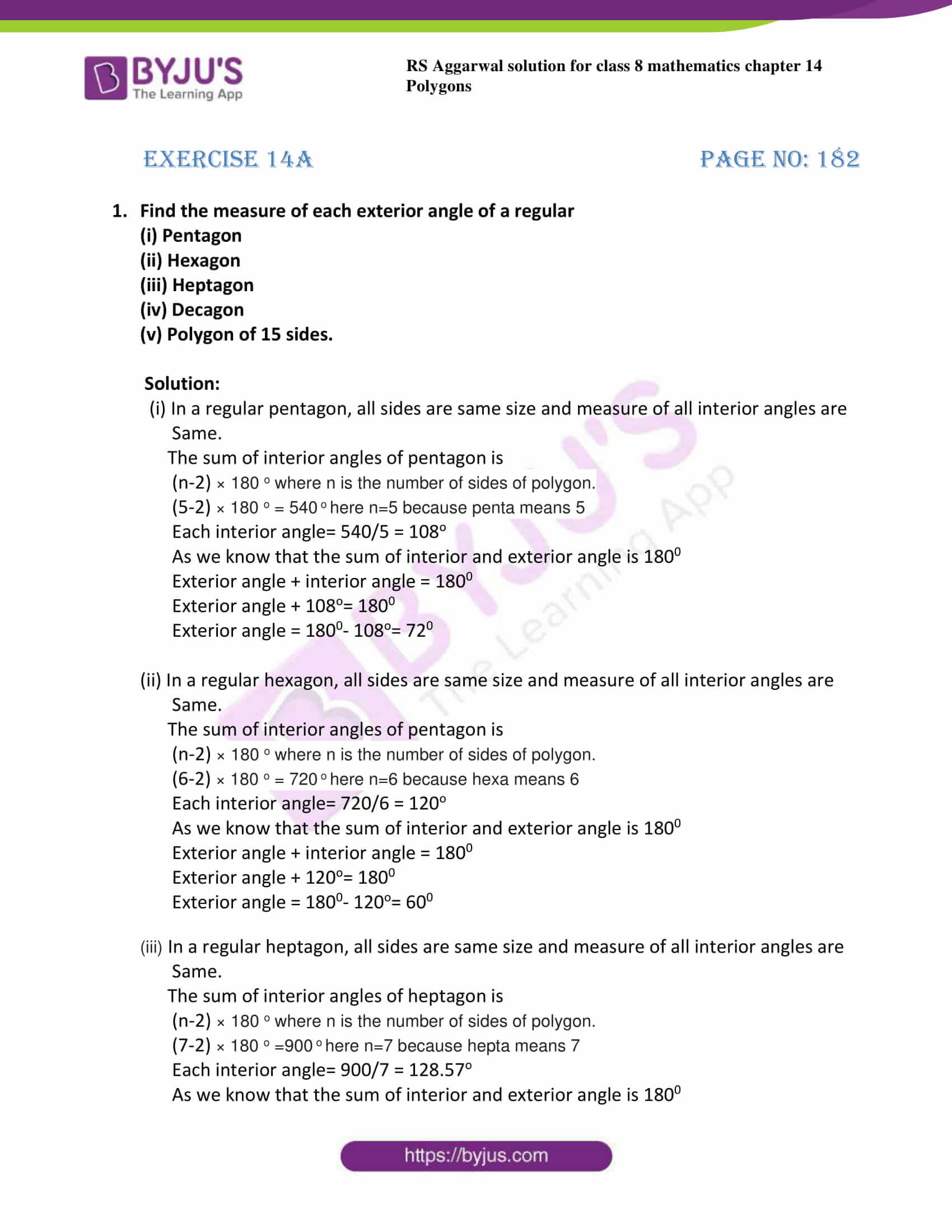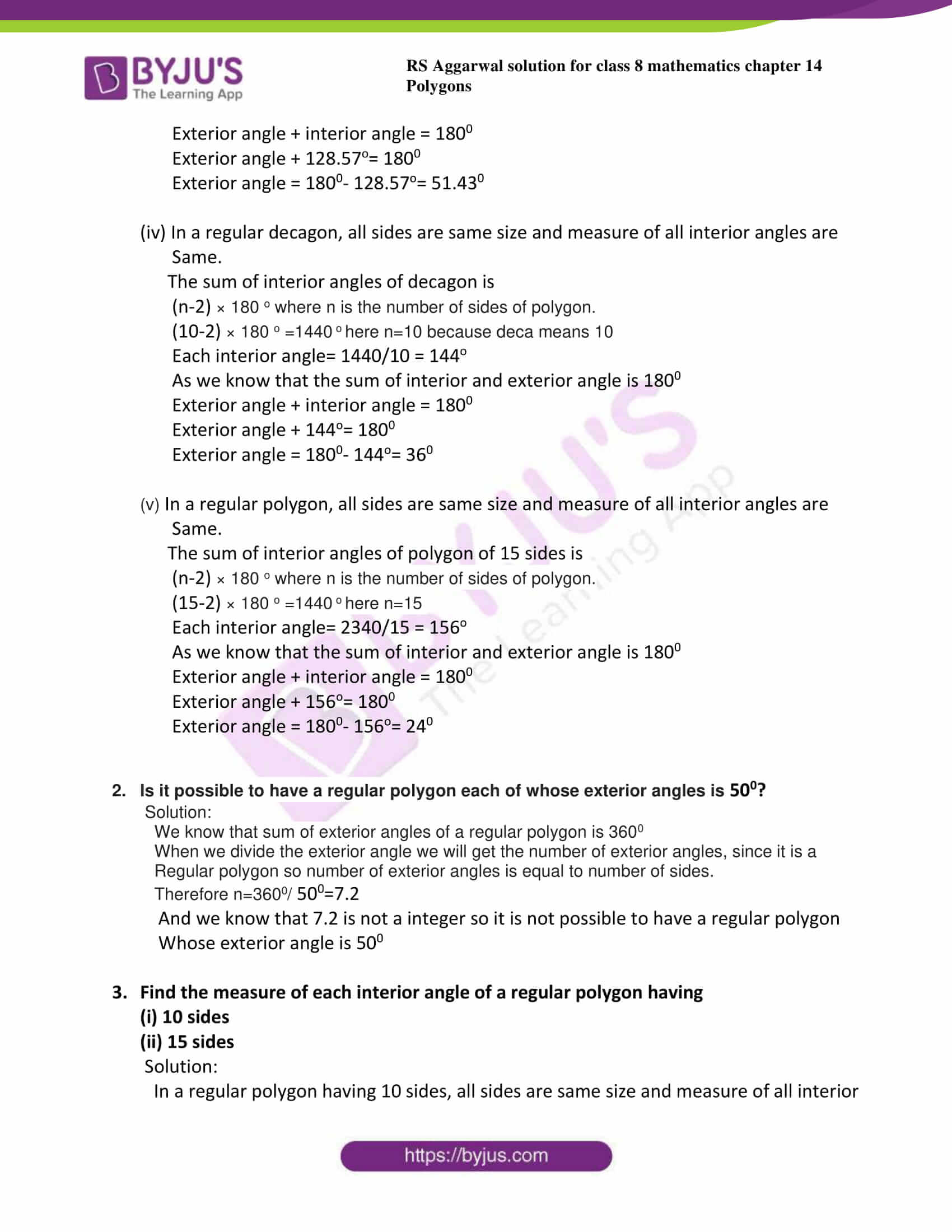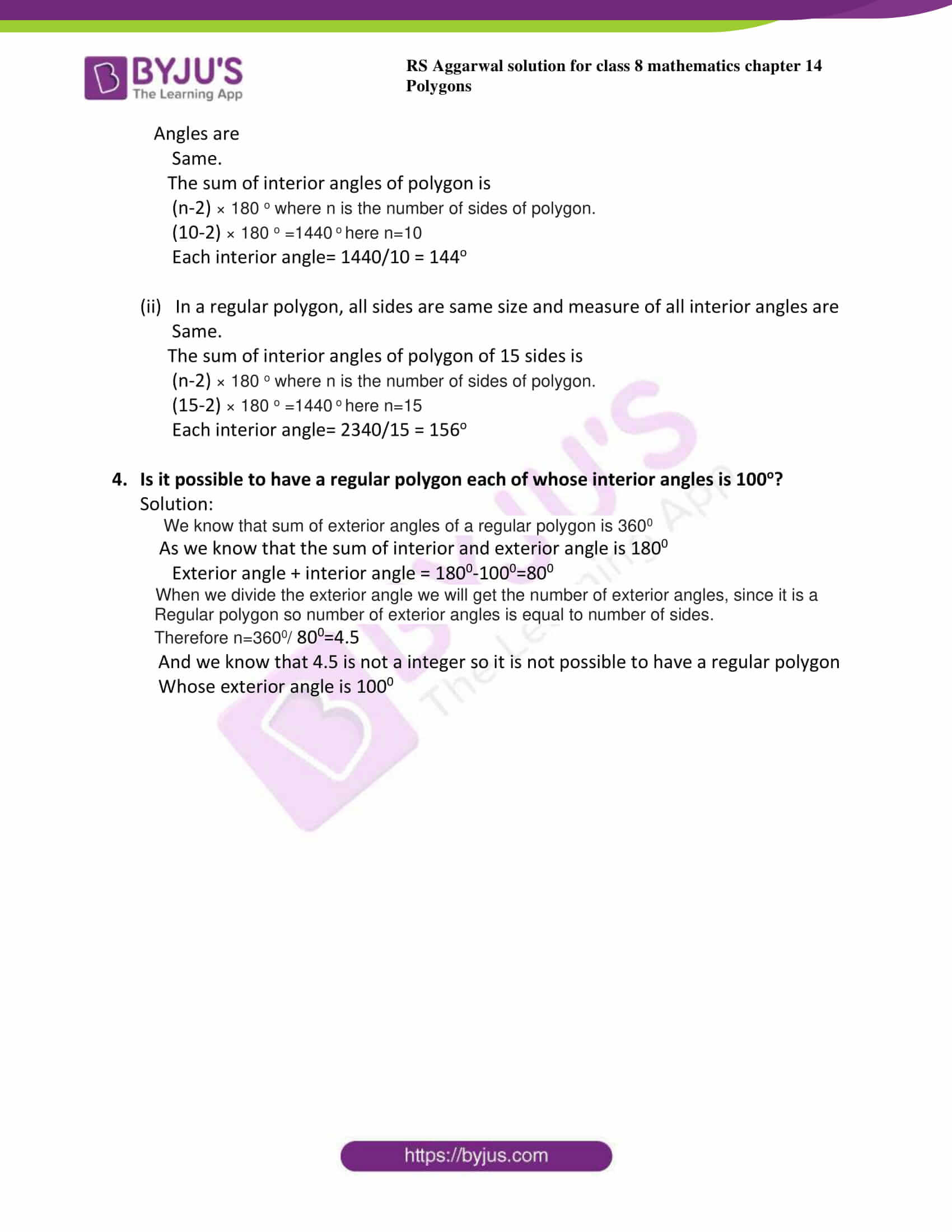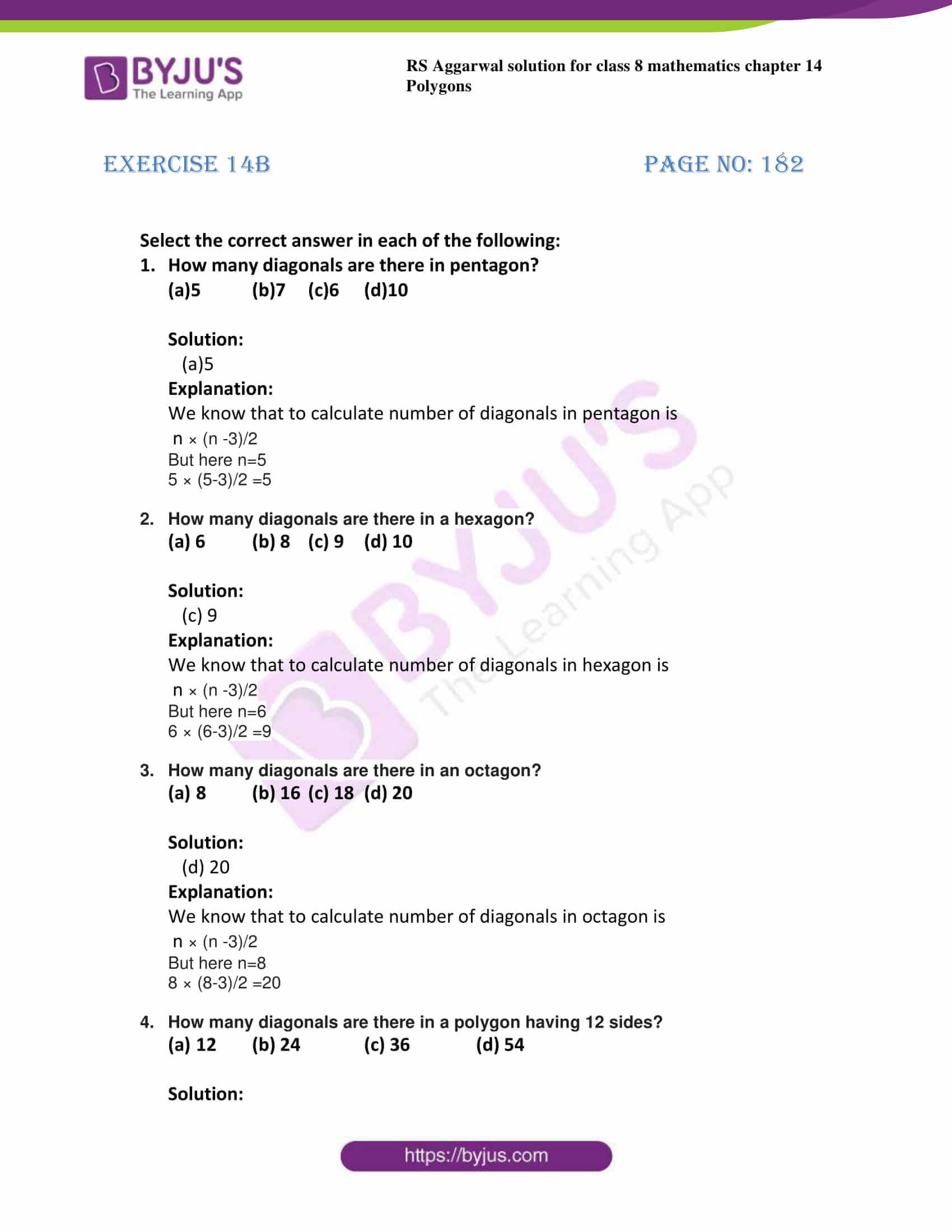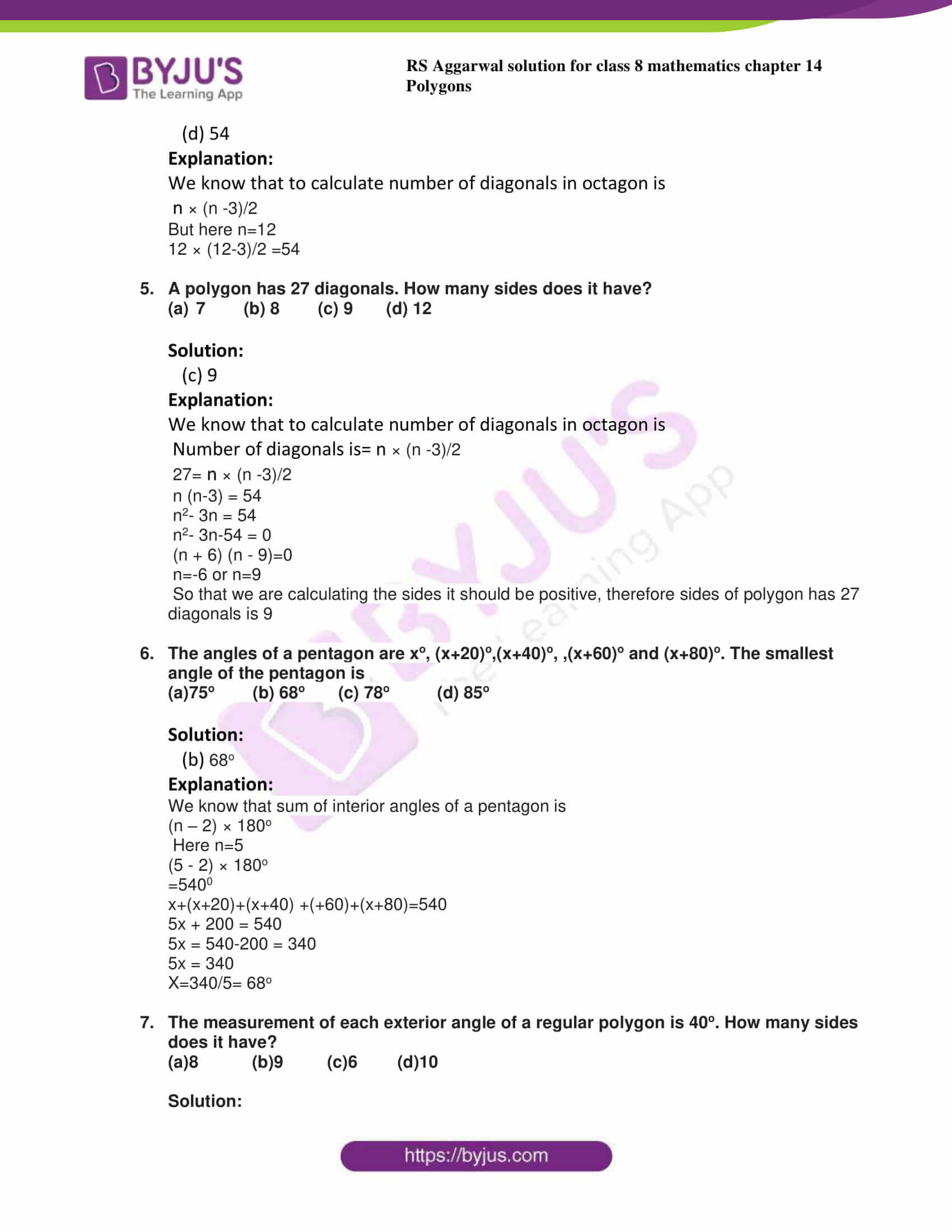## Exercise 14A

1. Find the measure of each exterior angle of a regular

(i) Pentagon

(ii) Hexagon

(iii) Heptagon

(iv) Decagon

(v) Polygon of 15 sides.

Solution:

(i) In a regular pentagon, all sides are same size and measure of all interior angles are

Same.

The sum of interior angles of pentagon is

(n-2) × 180 o where n is the number of sides of polygon.

(5-2) × 180 o = 540 o here n=5 because penta means 5

Each interior angle= 540/5 = 108o

As we know that the sum of interior and exterior angle is 1800

Exterior angle + interior angle = 1800

Exterior angle + 108o= 1800

Exterior angle = 1800– 108o= 720

(ii) In a regular hexagon, all sides are same size and measure of all interior angles are

Same.

The sum of interior angles of pentagon is

(n-2) × 180 o where n is the number of sides of polygon.

(6-2) × 180 o = 720 o here n=6 because hexa means 6

Each interior angle= 720/6 = 120o

As we know that the sum of interior and exterior angle is 1800

Exterior angle + interior angle = 1800

Exterior angle + 120o= 1800

Exterior angle = 1800– 120o= 600

(iii) In a regular heptagon, all sides are same size and measure of all interior angles are

Same.

The sum of interior angles of heptagon is

(n-2) × 180 o where n is the number of sides of polygon.

(7-2) × 180 o =900 o here n=7 because hepta means 7

Each interior angle= 900/7 = 128.57o

As we know that the sum of interior and exterior angle is 1800

Exterior angle + interior angle = 1800

Exterior angle + 128.57o= 1800

Exterior angle = 1800– 128.57o= 51.430

(iv) In a regular decagon, all sides are same size and measure of all interior angles are

Same.

The sum of interior angles of decagon is

(n-2) × 180 o where n is the number of sides of polygon.

(10-2) × 180 o =1440 o here n=10 because deca means 10

Each interior angle= 1440/10 = 144o

As we know that the sum of interior and exterior angle is 1800

Exterior angle + interior angle = 1800

Exterior angle + 144o= 1800

Exterior angle = 1800– 144o= 360

(v) In a regular polygon, all sides are same size and measure of all interior angles are

Same.

The sum of interior angles of polygon of 15 sides is

(n-2) × 180 o where n is the number of sides of polygon.

(15-2) × 180 o =1440 o here n=15

Each interior angle= 2340/15 = 156o

As we know that the sum of interior and exterior angle is 1800

Exterior angle + interior angle = 1800

Exterior angle + 156o= 1800

Exterior angle = 1800– 156o= 240

2. Is it possible to have a regular polygon each of whose exterior angles is 500?

Solution:

We know that sum of exterior angles of a regular polygon is 3600

When we divide the exterior angle we will get the number of exterior angles, since it is a

Regular polygon so number of exterior angles is equal to number of sides.

Therefore n=3600/ 500=7.2

And we know that 7.2 is not a integer so it is not possible to have a regular polygon

Whose exterior angle is 500

3. Find the measure of each interior angle of a regular polygon having

(i) 10 sides

(ii) 15 sides

Solution:

In a regular polygon having 10 sides, all sides are same size and measure of all interior

Angles are

Same.

The sum of interior angles of polygon is

(n-2) × 180 o where n is the number of sides of polygon.

(10-2) × 180 o =1440 o here n=10

Each interior angle= 1440/10 = 144o

(ii) In a regular polygon, all sides are same size and measure of all interior angles are

Same.

The sum of interior angles of polygon of 15 sides is

(n-2) × 180 o where n is the number of sides of polygon.

(15-2) × 180 o =1440 o here n=15

Each interior angle= 2340/15 = 156o

4. Is it possible to have a regular polygon each of whose interior angles is 100o?

Solution:

We know that sum of exterior angles of a regular polygon is 3600

As we know that the sum of interior and exterior angle is 1800

Exterior angle + interior angle = 1800-1000=800

When we divide the exterior angle we will get the number of exterior angles, since it is a

Regular polygon so number of exterior angles is equal to number of sides.

Therefore n=3600/ 800=4.5

And we know that 4.5 is not a integer so it is not possible to have a regular polygon

Whose exterior angle is 1000

## Exercise 14B

Select the correct answer in each of the following:

1. How many diagonals are there in pentagon?

(a)5 (b)7 (c)6 (d)10

Solution:

(a)5

Explanation:

We know that to calculate number of diagonals in pentagon is

n × (n -3)/2

But here n=5

5 × (5-3)/2 =5

2. How many diagonals are there in a hexagon?

(a) 6 (b) 8 (c) 9 (d) 10

Solution:

(c) 9

Explanation:

We know that to calculate number of diagonals in hexagon is

n × (n -3)/2

But here n=6

6 × (6-3)/2 =9

3. How many diagonals are there in an octagon?
a)8 (b) 16 (c) 18 (d) 20

Solution:

(d) 20

Explanation:

We know that to calculate number of diagonals in octagon is

n × (n -3)/2

But here n=8

8 × (8-3)/2 =20

4. How many diagonals are there in a polygon having 12 sides?
a)12 (b) 24 (c) 36 (d) 54

Solution:

(d) 54

Explanation:

We know that to calculate number of diagonals in octagon is

n × (n -3)/2

But here n=12

12 × (12-3)/2 =54

5. A polygon has 27 diagonals. How many sides does it have?
a)7 (b) 8 (c) 9 (d) 12

Solution:

(c) 9

Explanation:

We know that to calculate number of diagonals in octagon is

Number of diagonals is= n × (n -3)/2

27= n × (n -3)/2

n (n-3) = 54

n2– 3n = 54

n2– 3n-54 = 0

(n + 6) (n – 9)=0

n=-6 or n=9

So that we are calculating the sides it should be positive, therefore sides of polygon has 27 diagonals is 9

6. The angles of a pentagon are xo, (x+20)o,(x+40)o, ,(x+60)o and (x+80)o. The smallest angle of the pentagon is

(a)75o (b) 68o (c) 78o (d) 85o

Solution:

(b) 68o

Explanation:

We know that sum of interior angles of a pentagon is

(n – 2) × 180o

Here n=5

(5 – 2) × 180o

=5400

x+(x+20)+(x+40) +(+60)+(x+80)=540

5x + 200 = 540

5x = 540-200 = 340

5x = 340

X=340/5= 68o

7. The measurement of each exterior angle of a regular polygon is 40o. How many sides does it have?

(a)8 (b)9 (c)6 (d)10

Solution:

(b)9

Explanation:

Given exterior angle= 40o

But we know that Number of sides = 360/ exterior angle

Number of sides = 360/40=9

## RS Aggarwal Solutions for Class 8 Maths Chapter 14- Polygons

Chapter 14, Polygons, contains 2 Exercises. RS Aggarwal Solutions given here contains the answers to all the questions present in these exercises. Let us have a look at some of the concepts that are being discussed in this chapter.

• Definition of curve
• Definition of open curve
• Definition of closed curve
• Definition of simple closed curve
• Definition of polygons
• Definition of regular polygon
• Definition of irregular polygon
• Exterior angle calculation
• Interior angle calculation
• Formula to calculate the sum of all exterior angles
• Formula to calculate the sum of all interior angles
• Formula to calculate the number of diagonals in a polygon of ‘n’ side

Exercise 14A

Exercise 14B

### Chapter Brief of RS Aggarwal Solutions for Class 8 Maths Chapter 14 – Polygons

The RS Aggarwal Solutions for Class 8 Maths Chapter 14 – Polygons deals with the definition of curves, types of curves, definition of polygons, and its types and also some of the formulae to calculate interior angle, exterior angle, number of diagonals. These formulae will help in calculating the angles, diagonals without drawing the figure.